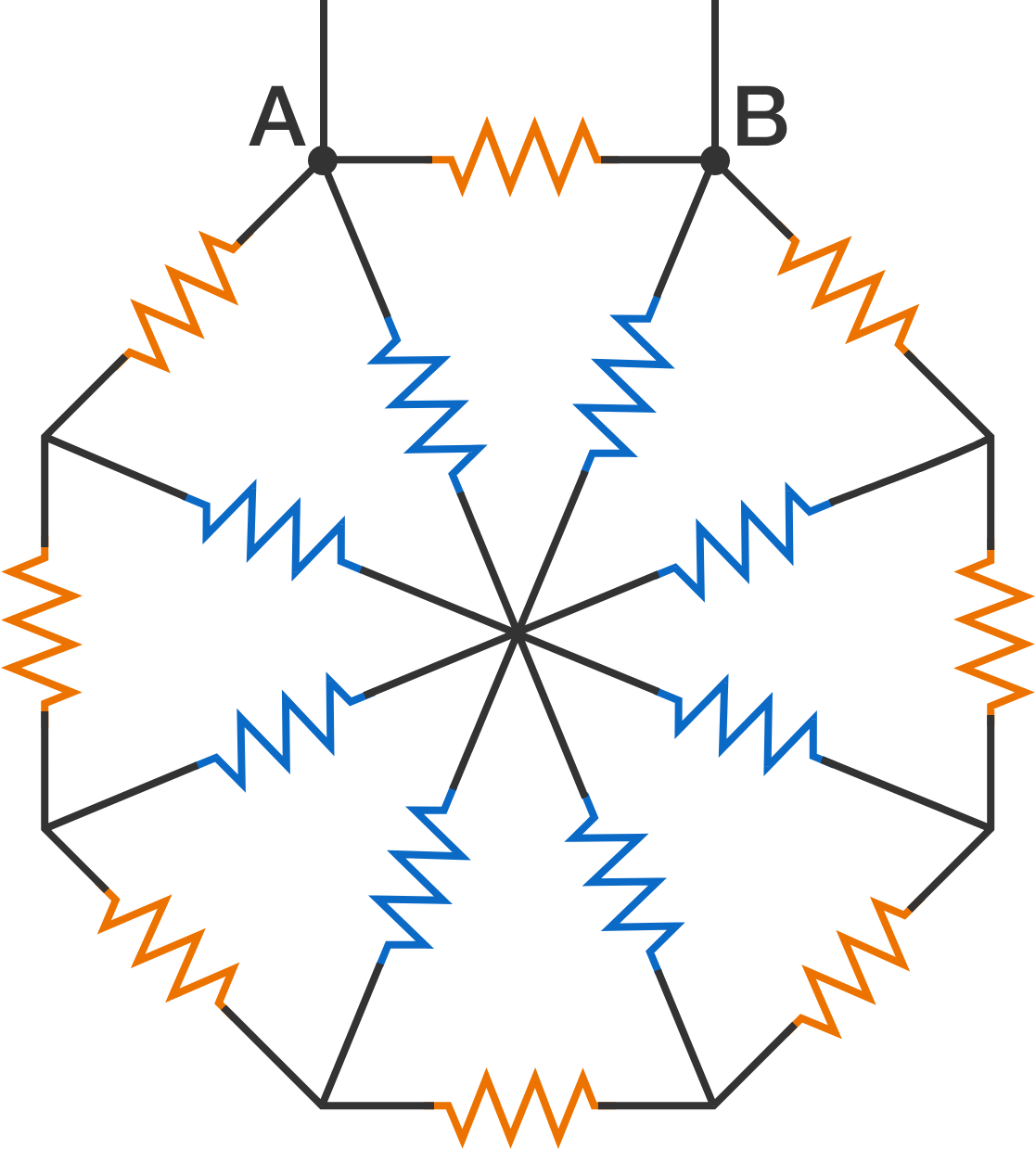# Octagonal Resistor System8 resistors (orange color) are connected to form a regular octagon. 8 more resistors (blue color) connect the vertices of the octagon to its center. All the 16 resistors are of resistance $\SI{420}{\ohm}$.

If the connecting wires have negligible resistance, calculate the equivalent resistance (in ohms, rounded to the nearest integer) between the terminals $A$ and $B$.

×

Problem Loading...

Note Loading...

Set Loading...